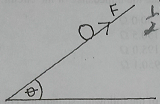mycollegehive
The diagram above illustrates a ball of mass m sliding dow...
waec-2020 ssce-exam-2020 waec-physics-2020 physics0The diagram above illustrates a ball of mass m sliding down a plank inclined at an angle of θ to the horizontal. The kinetic friction between the ball and the plank is F and acceleration of free fall is g

The net force on the ball along the plank is

A. mgsinθ + F

B. F - mgcosθ

C. mgsinθ - F

D. F + mgcosθ

232 viewsShareFollowUniversity of Lagos Nigeria
31 August 2020Jace,
C
3 likes, 0 dislikes

How to insert math formulas/equations### Related Tags

waec-2020

8 followers

273 questionsssce-exam-2020

6 followers

273 questionswaec-physics-2020

6 followers

48 questions